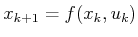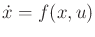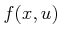# 13. Differential Models

This chapter provides a continuous-time counterpart to the state transition equation,, which was crucial in Chapter 2. On a continuous state space,(assumed to be a smooth manifold), it will be defined as, which intentionally looks similar to the discrete version. It will still be referred to as a state transition equation. It will also be called a system (short for control system), which is a term used in control theory. There are no obstacle regions in this chapter. Obstacles will appear again when planning algorithms are covered in Chapter 14. In continuous time, the state transition functionyields a velocity as opposed to the next state. Since the transitions are no longer discrete, it does not make sense to talk about a next'' state. Future states that satisfy the differential constraints are obtained by integration of the velocity. Therefore, it is natural to specify only velocities. This relies on the notions of tangent spaces and vector fields, as covered in Section 8.3.

This chapter presents many example models that can be used in the planning algorithms of Chapter 14. Section 13.1 develops differential constraints for the case in whichis the C-space of one or more bodies. These constraints commonly occur for wheeled vehicles (e.g., a car cannot move sideways). To represent dynamics, constraints on acceleration are needed. Section 13.2 therefore introduces the phase space, which enables any problem with dynamics to be expressed as velocity constraints on an enlarged state space. This collapses the higher order derivatives down to being only first-order, but it comes at the cost of increasing the dimension of the state space. Section 13.3 introduces the basics of Newton-Euler mechanics and concludes with expressing the dynamics of a free-floating rigid body. Section 13.4 introduces some concepts from advanced mechanics, including the Lagrangian and Hamiltonian. It also provides a model of the dynamics of a kinematic chain of bodies, which applies to typical robot manipulators. Section 13.5 introduces differential models that have more than one decision maker.

Subsections
Steven M LaValle 2020-08-14